pcbStack

Single-feed or multifeed PCB antenna

Description

The pcbStack object is a single-feed or multi-feed printed circuit board (PCB) antenna. The pcbStack object can be used

• To create single-layer, multilayer metal, or metal-dielectric substrate antennas

• To create an arbitrary number of feeds and vias in an antenna

• To create a PCB antenna with Antenna Toolbox™ antenna catalog elements

• To convert antenna array elements to PCB stack

Note

To generate a Gerber file, a substrate layer is required. Use the Substrate property to create this layer in the PCB antenna. For more information, see Stack Conversion

Creation

Description

pcbant = pcbStack creates an air-filled single-feed PCB with two metal layers.

example

pcbant = pcbStack(Name,Value) sets Properties using name-value pairs. For example, pcbStack('FeedDiameter', 2.000e-04) creates a PCB antenna with a feed diameter of 2.000e-04 meters. You can specify multiple name-value pairs. Enclose each property name in quotes creates a PCB antenna, with additional properties specified by one or more name-value pair arguments. Properties not specified retain their default values.

example

pcbant = pcbStack(ant) converts any 2-D or 2.5-D antenna from the antenna catalog into a PCB antenna for further modeling and analysis. You can also use antenna array objects from the antenna array catalog elements them convert it into PCB antennas.

Properties

expand all

Name of PCB antenna, specified a character vector.

Example: 'Name','PCBPatch'

Data Types: char | string

Revision details of PCB antenna design, specified as a character vector.

Example: 'Revision','2.0'

Data Types: char | string

Shape of PC board, specified as an object. The shape can be a rectangle or a polygon.

Example: 'BoardShape',antenna.Polygon

Thickness of PC board, specified as a positive scalar.

Example: 'BoardThickness',0.02000

Data Types: double

Metal and dielectric layers, specified a cell array of metal layer shapes and dielectric. You can specify one metal shape or one dielectric per layer starting with the top layer and proceeding down.

Data Types: cell

Feed locations for PCB antenna in Cartesian coordinates, specified as N -by-3 or N-by-4 array. You can place feed inside the board or at the edge of the board. The arrays translate to the following:

• N -by-3 – [x, y, Layer]

• N-by-4 – [x, y, SigLayer, GndLayer]

Example: 'FeedLocations',[-0.0187 0 1 2]

Data Types: double

Center pin diameter of feed connector, specified as a positive scalar in meters.

Example: 'FeedDiameter',2.000e-04

Data Types: double

Electrical short locations for antenna in Cartesian coordinates, specified as a real vector of size M-by-4 array. The arrays translate to the following:

• M-by-4 – [x, y, SigLayer, GndLayer]

Example: 'ViaLocations',[0 -0.025 1 2]

Data Types: double

Electrical shorting pin diameter between metal layers, specified a positive scalar in meters.

Data Types: double

Magnitude voltage applied at the feeds, specified as a positive scalar in volts.

Example: 'FeedVoltage',2

Data Types: double

Model for approximating feed and via, specified as one of the following:

• 'strip' – A rectangular strip approximation to the feed or via cylinder. This approximation is the simplest and results in a small mesh.

• 'square' – A 4-sided polyhedron approximation to the feed or via cylinder.

• 'hexagon' – A 6-sided polyhedron approximation to the feed or via cylinder.

• 'octagon' – A 8-sided polyhedron approximation to the feed or via cylinder.

Example: 'FeedViaModel','octagon'

Data Types: char | string

Excitation phase at each feed, specified as a real vector in degrees.

Example: 'FeedPhase',2

Data Types: double

Type of the metal used as a conductor, specified as a metal material object. You can choose any metal from the MetalCatalog or specify a metal of your choice. For more information, see metal. For more information on metal conductor meshing, see Meshing.

Example: m = metal('Copper'); 'Conductor',m

Example: m = metal('Copper'); ant.Conductor = m

Lumped elements added to the antenna feed, specified as a lumped element object handle. For more information, see lumpedElement.

Example: 'Load',lumpedelement. lumpedelement is the object handle for the load created using lumpedElement.

Tilt angle of the antenna, specified as a scalar or vector with each element unit in degrees. For more information, see Rotate Antennas and Arrays.

Example: 'Tilt',90

Example: ant.Tilt = 90

Example: 'Tilt',[90 90],'TiltAxis',[0 1 0;0 1 1] tilts the antenna at 90 degrees about the two axes defined by the vectors.

Note

The wireStack antenna object only accepts the dot method to change its properties.

Data Types: double

Tilt axis of the antenna, specified as:

• Three-element vector of Cartesian coordinates in meters. In this case, each coordinate in the vector starts at the origin and lies along the specified points on the X-, Y-, and Z-axes.

• Two points in space, each specified as three-element vectors of Cartesian coordinates. In this case, the antenna rotates around the line joining the two points in space.

• A string input describing simple rotations around one of the principal axes, 'X', 'Y', or 'Z'.

Example: 'TiltAxis',[0 1 0]

Example: 'TiltAxis',[0 0 0;0 1 0]

Example: ant.TiltAxis = 'Z'

Note

The wireStack antenna object only accepts the dot method to change its properties.

Data Types: double

Object Functions

 show Display antenna or array structure; display shape as filled patch array Create array of PCB stack objects axialRatio Axial ratio of antenna beamwidth Beamwidth of antenna charge Charge distribution on metal or dielectric antenna or array surface current Current distribution on metal or dielectric antenna or array surface efficiency Radiation efficiency of antenna EHfields Electric and magnetic fields of antennas; Embedded electric and magnetic fields of antenna element in arrays impedance Input impedance of antenna; scan impedance of array info Display information about antenna or array layout Display array or PCB stack layout mesh Mesh properties of metal or dielectric antenna or array structure meshconfig Change mesh mode of antenna structure pattern Radiation pattern and phase of antenna or array; Embedded pattern of antenna element in array patternAzimuth Azimuth pattern of antenna or array patternElevation Elevation pattern of antenna or array returnLoss Return loss of antenna; scan return loss of array sparameters Calculate S-parameter for antenna and antenna array objects vswr Voltage standing wave ratio of antenna plot Plot boundary of shape

Examples

collapse all

Setup parameters.

vp  = physconst('lightspeed');
f   = 850e6;
lambda = vp./f;

L = 0.15;
W = 1.5*L;
stripL = L;
gapx = .015;
gapy = .01;
r1 = antenna.Rectangle('Center',[0,0],'Length',L,'Width',W,'Center',[lambda*0.35,0]);
r2 = antenna.Rectangle('Center',[0,0],'Length',L,'Width',W,'Center',[-lambda*0.35,0]);
r3 = antenna.Rectangle('Length',0.5*lambda,'Width',0.02*lambda,'NumPoints',2);
s = r1 + r2 + r3;
figure
show(s)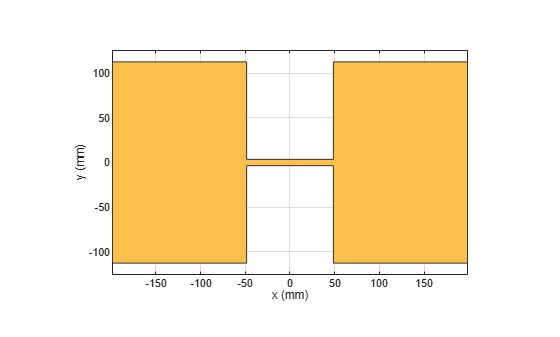Assign the radiator shape to pcbStack and make the changes to the board shape and feed diameter properties.

boardShape = antenna.Rectangle('Length',0.6,'Width',0.3);
p = pcbStack;
p.BoardShape = boardShape;
p.Layers = {s};
p.FeedDiameter = .02*lambda/2;
p.FeedLocations = [0 0 1];
figure
show(p)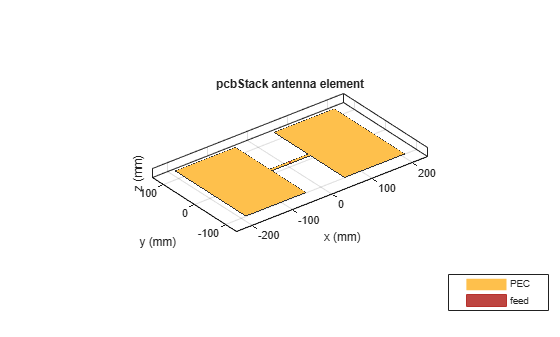Analyze the impedance of the antenna. Effect of the end-loading should result in the series resonance to be pushed lower in the band.

figure
impedance(p,linspace(200e6,1e9,51))Create a pcb stack antenna with 2 mm dielectric thickness at the radiator and air below it. Display the structure.

p = pcbStack;
d1 = dielectric('FR4');
d1.Thickness = 2e-3;
d2 = dielectric('Air');
d2.Thickness = 8e-3;
p.Layers = {p.Layers{1},d1,d2,p.Layers{2}};
p.FeedLocations(3:4) = [1 4];
show(p)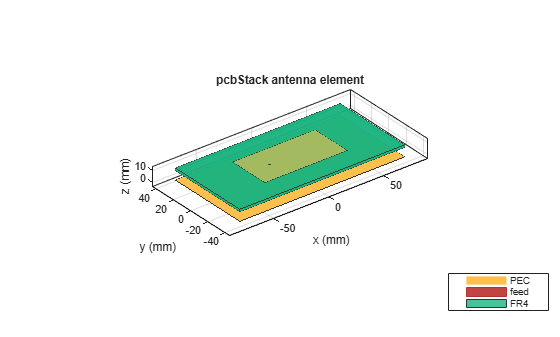Create a PCB stack antenna from reflector backed bowtie.

b = design(bowtieRounded,1e9);
b.Tilt = 90
b =
bowtieRounded with properties:

Length: 0.0959
FlareAngle: 90
Conductor: [1x1 metal]
Tilt: 90
TiltAxis: [1 0 0]

b.TiltAxis = [0 1 0];
r = reflector('Exciter',b);
p = pcbStack(r);

Plot the directivity pattern of the antenna at 1 GHz.

pattern(p,1e9);Create a coplanar inverted F antenna.

fco = invertedFcoplanar('Height',14e-3,'GroundPlaneLength', 100e-3,  ...
'GroundPlaneWidth', 100e-3);

Use this antenna to create a pcbStack object.

p = pcbStack(fco);

Create a circular microstrip patch.

p = patchMicrostripCircular;
d = dielectric;
d.EpsilonR = 4.4;
p.Height = 1.6e-3;
p.Substrate = d;
p.GroundPlaneLength = 3*.0256;
p.GroundPlaneWidth = 3*.0256;
p.FeedOffset = [.0116 0];

Create a PCB circular microstrip patch using pcbStack.

pb = pcbStack(p);
pb.FeedDiameter = 1.27e-3;
pb.ViaLocations = [0 pb.FeedLocations(1)/1.1 1 3];
figure
show(pb)C = SMA_Jack_Cinch;
O = PCBServices.MayhewWriter;
O.Filename = 'Microstrip circular patch-9a';
Am = PCBWriter(pb,O,C);
gerberWrite(Am)

Images using Mayhew Labs 3-D Viewer.Create a coplanar inverted-F antenna.

fco = invertedFcoplanar('Height',14e-3,'GroundPlaneLength', 100e-3,  ...
'GroundPlaneWidth', 100e-3);

Create a linear array with inverted-F antenna as its elements.

la = linearArray;
la.Element = fco;
la.NumElements = 4;

Use this antenna array to create the PCB antenna.

p = pcbStack(la);

Create a dipole antenna object and linearArray antenna array object. In the linearArray antenna object, leave the Element property set to its default value of dipole. Set the ElementSpacing property to 4."

d1 = dipole;
d2 = linearArray('ElementSpacing', 4);

To set the Z-coordinate of pcbStack antenna object to zero, rotate the dipole and linear dipole array around 90 degrees using the Tilt property. Then set the TiltAxis property to [ 0 -1 0 ] for dipole and linear dipole array antennas.

d1.Tilt = 90;
d2.Element.Tilt = 90;
d1.TiltAxis = [0 -1 0];
d2.Element.TiltAxis = [0 -1 0];

Create and view PCB stack antenna created using the dipole antenna object.

p1 = pcbStack(d1);
show(p1)Create and view PCB stack antenna using the linearArray antenna array object.

p2 = pcbStack(d2);
show(p2)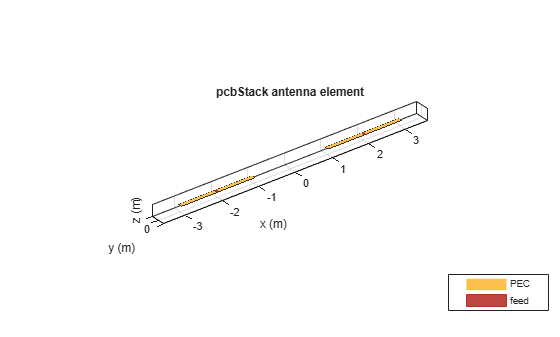Create a circular microstrip patch antenna.

ant = design(patchMicrostripCircular,3e9);
ant.Substrate = dielectric( 'FR4' );
show(ant)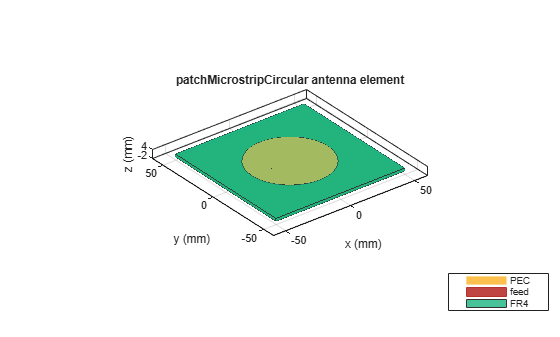c = antenna.Circle;
show(c)c.NumPoints = 6;
figure
show(c)Create the PCB stack using the vertices derived from the circle shape.

v = getShapeVertices(c);
cp = antenna.Polygon( 'Vertices' ,v);
pb = pcbStack(ant);
pb.Layers{3} = cp;
pb.BoardShape = cp;
show(pb)
axis equal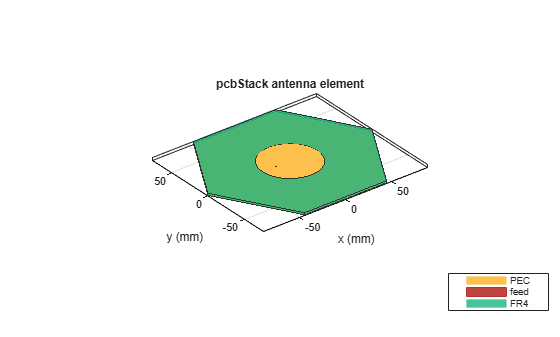Balanis, C. A. Antenna Theory. Analysis and Design. 3rd Ed. Hoboken, NJ: John Wiley & Sons, 2005.

 Stutzman, W. L. and Gary A. Thiele. Antenna Theory and Design. 3rd Ed. River Street, NJ: John Wiley & Sons, 2013.